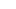December 19, 2016

https://youtu.be/uGSkNh-oEgE

Relational operators:

< Less Than

> Greater Than

== Equal To

<= Less than or equal to

>= Greater Than or equal to

!= not equal to

These operators return a boolean value true or false. They report on the relationship of the two values that surround...

December 15, 2016

https://youtu.be/YQgFQcLOLBY

The if structure explains the processing in the following:

Input > Processing > Output

Simple

if this then that

decisions

Syntax:

if this :

Do that

Example:

if x < 9:

print(“x is less than 9.”)

Mak...

December 13, 2016

https://youtu.be/7Bwtj-NwOTE

Casting input to get what you want:

If you want numeric data from a string, you must assume that the user gave you what you want:

print(“In 5 years you will be %i years old.” % int(age)+5 )

If you want (bad idea) y...

December 9, 2016

https://youtu.be/YelkDnk97HQ

Input -> Processing -> Output

Input is defined in this context as data the user gives to the program. The program then uses that data in some way - manipulates it or just stores it in memory. Finally th...

December 7, 2016

https://youtu.be/xQAax3rL92o

Basic Math in Python works as you expect it will:

- subtracts

* multiplies

/ divides

x**y raises x to the exponent y

( ) work like they should

% modulo - gives remainder of division

Also works as expected

PEMDAS:

1. Parentheses

2. Exponent...

December 5, 2016

https://youtu.be/GYHyZxX3oNM

Some of my users gave me grief on the brevity of my last two videos so here's one that pushes the limit - running about 10 minutes.

Literals are any values you can see in the code itself.

For instance,

print("Hello, World")

The string "Hello, W...

###### Featured Post